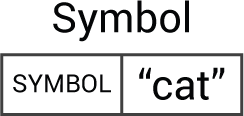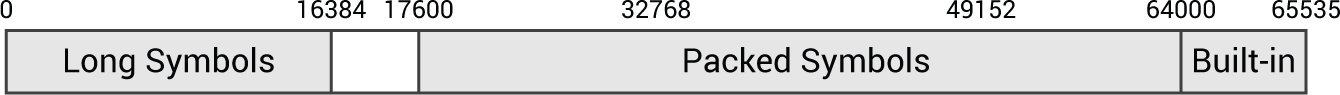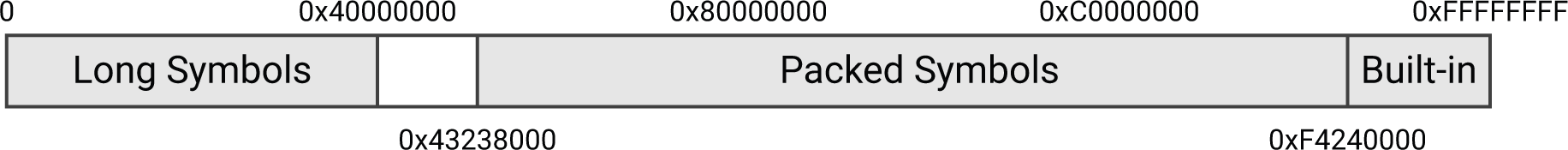## Symbols

This page describes the implementation of symbols as of uLisp Release 4.0.

The following description refers to the 16-bit versions of uLisp. The 32-bit versions are essentially the same, except that objects consist of two 32-bit cells.

6th February 2023: Improved the explanation of how symbols are represented.

### Symbol object

A symbol object consists of a SYMBOL identifier in the left-hand cell, and the symbol or a pointer to the symbol in the right-hand cell:#### Creating a symbol

You would create a symbol object called sym as follows:

```object *sym = myalloc();
sym->type = SYMBOL;
sym->name = name;```

where name is the 16-bit symbol name representing the symbol. This has type symbol_t.

#### Representing symbols - 16-bit platforms

The most general type of symbol is a pointer to a string, represented by a linked list of Lisp objects. Because Lisp objects are aligned on a four-byte boundary, pointer addresses must be an exact multiple of four, and we can take advantage of this redundancy to use the remaining unused 16-bit numbers to provide a more compact representation for commonly used symbols.

The number space is transformed using a twist() macro to create the actual symbol name field, and an untwist() macro is provided to perform the reverse transformation:

```#define twist(x)           ((uint16_t)((x)<<2) | ((x)>>14 & 0x03))
#define untwist(x)         (((x)>>2 & 0x3FFF) | ((x) & 0x03)<<14)```

The 16-bit cell number space is used to represent three different types of symbols:

• Long symbols, for arbitrary symbols with any number of characters.
• Packed symbols, for user-defined symbols of up to three characters from a 40-character set.
• Built-in symbols, for the functions and other symbols provided in the uLisp language.

The following diagram shows how the untwisted number space is allocated to each type of symbol:• Values 0 to 16383 represent long symbols.
• Values 16384 to 17599 are not used.
• Values 17600 to 63999 represent packed symbols of up to three characters.
• Values 64000 to 65535 represent the built-in symbols, allowing for a maximum of 1536 built-in symbols.

#### Representing symbols - 32-bit platforms

On 32-bit platforms the twist() and untwist() macros are:

```#define twist(x)           ((uint32_t)((x)<<2) | ((x)>>30 & 0x03))
#define untwist(x)         (((x)>>2 & 0x3FFFFFFF) | ((x) & 0x03)<<30)```

The 32-bit cell number space is used to represent three different types of symbols:

• Long symbols, for arbitrary symbols with any number of characters.
• Packed symbols, for user-defined symbols of up to six characters from a 40-character set.
• Built-in symbols, for the functions and other symbols provided in the uLisp language.

The following diagram shows how the untwisted number space is allocated to each type of symbol:• Values 0 to 0x40000000 represent long symbols.
• Values 0x40000000 to 0x43237FFF are not used.
• Values 0x43238000 to 0xF423FFFF represent packed symbols of up to six characters.
• Values 0xF4240000 to 0xFFFFFFFF represent the built-in symbols, allowing for a maximum of 0x0BDC0000 built-in symbols.

The three symbol types are described in greater detail in the following sections:

### Long symbols

There is no symbol table as in previous versions of uLisp. Instead, long symbols are represented using the same representation as uLisp strings.

The symbol name in the right-hand cell is a pointer to the start of the symbol, and pairs of characters are stored in a linked list of cells. For example, here is the representation of the symbol hello:Because objects are aligned on a 4-byte boundary, the bottom two bits of the symbol name will be zero.

### Built-in symbols

The built-in symbols are defined by a C enum, with type builtin_t. This is useful as the compiler will give a warning when a symbol_t type is used where a builtin_t type is expected, although they are actually both 16-bit integers.

The built-in symbols are the indices into the symbol lookup table, and have values from 1 up to about 180, depending on the platform.

The builtin() function converts the symbol's name cell to a built-in index:

```builtin_t builtin (symbol_t name) {
return (builtin_t)(untwist(name) - BUILTINS);
}```

The reverse function sym() converts a built-in index into a symbol name:

```symbol_t sym (builtin_t x) {
return twist(x + BUILTINS);
}```

### Packed symbols

Packed symbols are an optional additional representation for symbols, to allow you to save RAM by using short symbol names. The following description applies to the three-character packed symbols used on 8/16-bit platforms. The same approach is used on 32-bit platforms, except that up to six characters can be packed.

A three-character long symbol such as "len" takes three objects; ie 12 bytes. Representing it as a packed symbol takes only one object; ie 4 bytes.

Values 17600 to 63999 represent packed symbols of up to three characters.

As in previous versions of uLisp, RAM is saved by packing short symbols of up to three characters into a single 16-bit value using radix-40 encoding, based on a character set of 40 characters.

The symbol can be represented in packed format if:

• It consists of up to three characters.
• Each character is 0 to 9, a to z, \$, *, or -.
• The first character is not a digit.

Here are some examples of packed symbols and their values:

• a = 17600
• z00 = 57641
• \$\$\$ = 63999

Note that three-character symbols starting with a digit are valid in Lisp, but they are represented in uLisp as long symbols as their packed representation would overlap with the long symbols.

The routine valid40() checks whether a symbol can be represented as a valid packed symbol:

```bool valid40 (char *buffer) {
}```

Characters are packed by pack40():

```int pack40 (char *buffer) {
}```

This in turn calls toradix40() to convert the characters in the character set to values between 0 and 39:

```int8_t toradix40 (char ch) {
if (ch == 0) return 0;
if (ch >= '0' && ch <= '9') return ch-'0'+1;
if (ch == '-') return 37; if (ch == '*') return 38; if (ch == '\$') return 39;
ch = ch | 0x20;
if (ch >= 'a' && ch <= 'z') return ch-'a'+11;
return -1; // Invalid
}```

The corresponding routine fromradix40() converts a number from 0 to 39 to the corresponding character in the character set:

```char fromradix40 (int n) {
if (n >= 1 && n <= 10) return '0'+n-1;
if (n >= 11 && n <= 36) return 'a'+n-11;
if (n == 37) return '-'; if (n == 38) return '*'; if (n == 39) return '\$';
return 0;
}```

### Testing the type of a symbol

The following functions allow you to test what type of symbol a symbol name field represents.

The function builtinp() checks whether a symbol name represents a builtin symbol:

```bool builtinp (symbol_t name) {
return (untwist(name) > BUILTINS && untwist(name) < ENDFUNCTIONS+BUILTINS);
}```

The macro longsymbolp() tests the bottom two bits of the name to determine if the symbol is a long symbol:

`#define longsymbolp(x)     (((x)->name & 0x03) == 0)`

Previous: Objects

Next: Built-in symbols